冒泡排序图解冒泡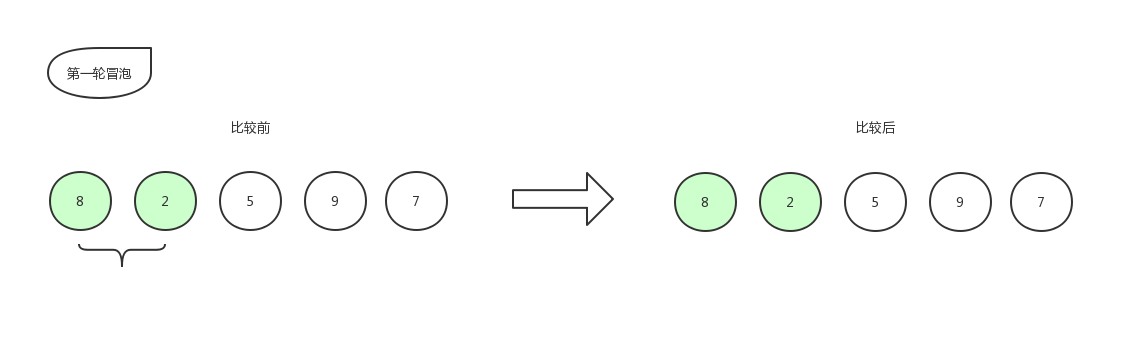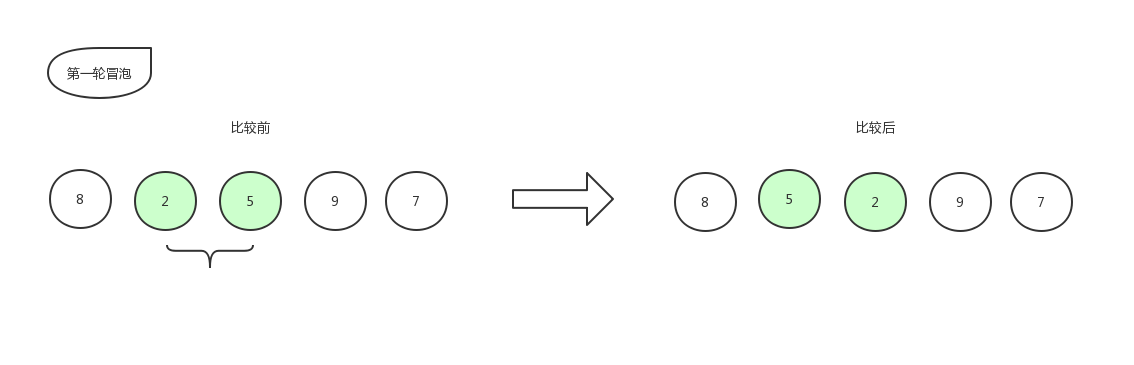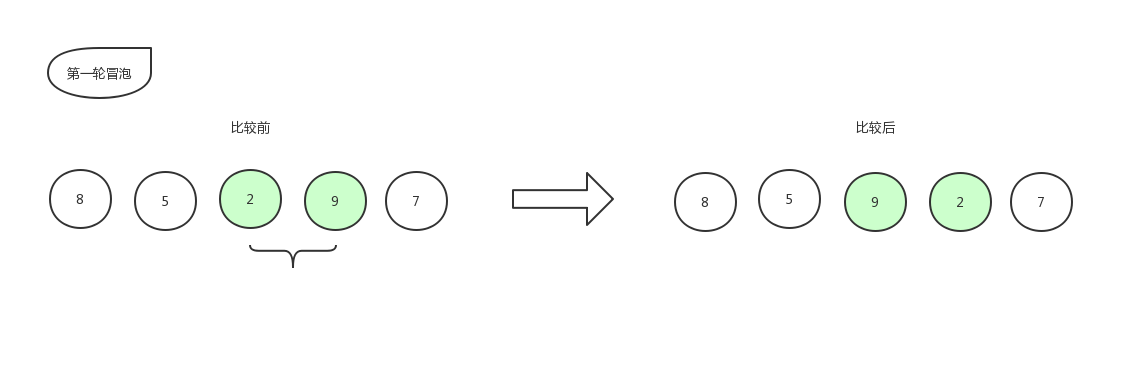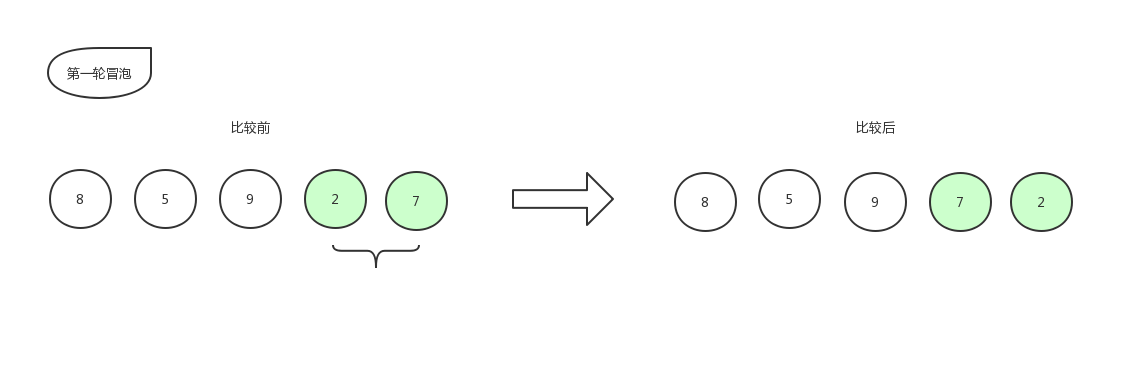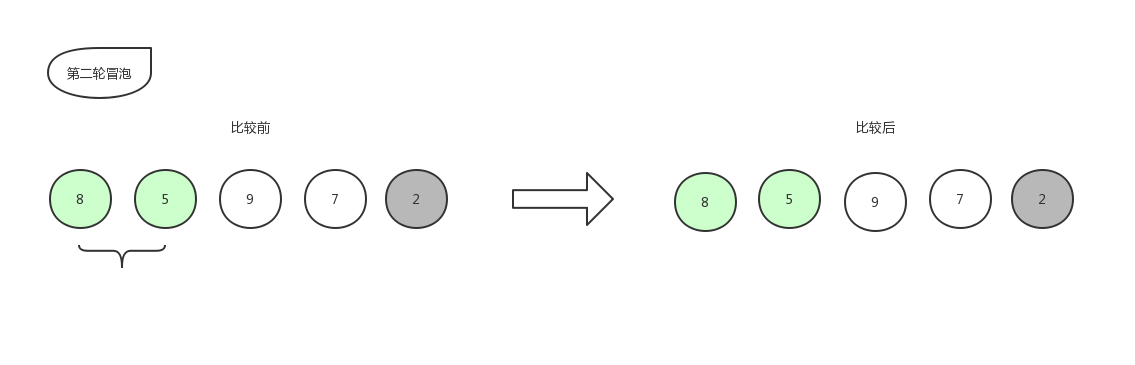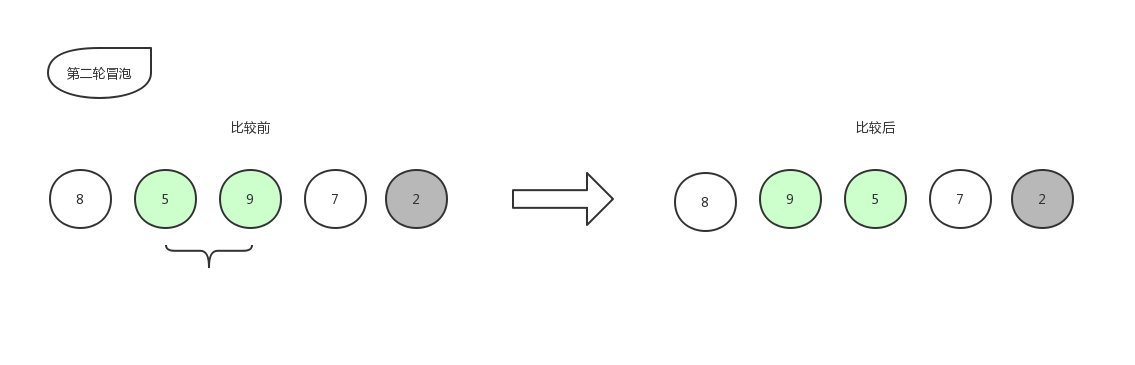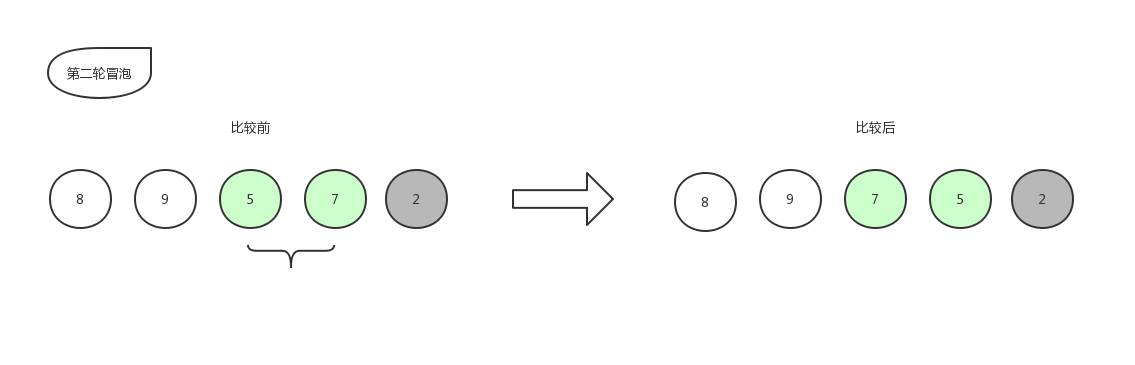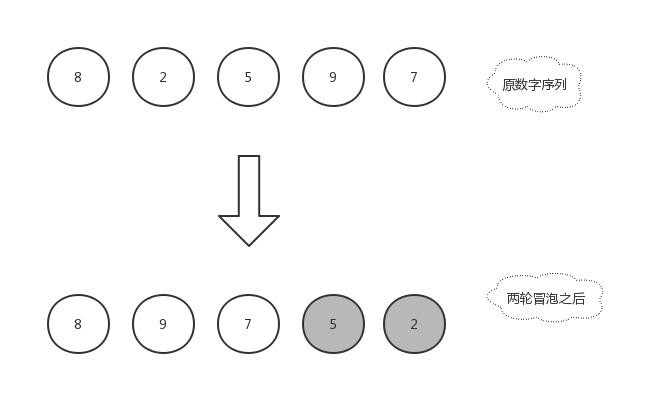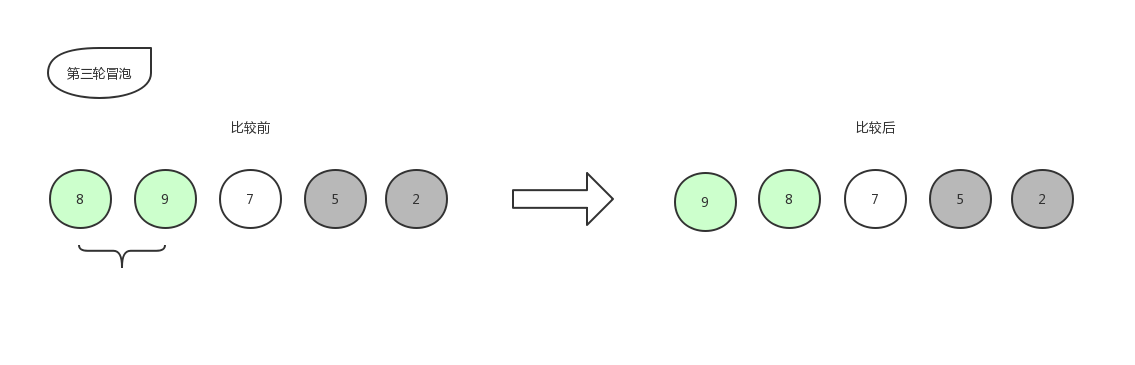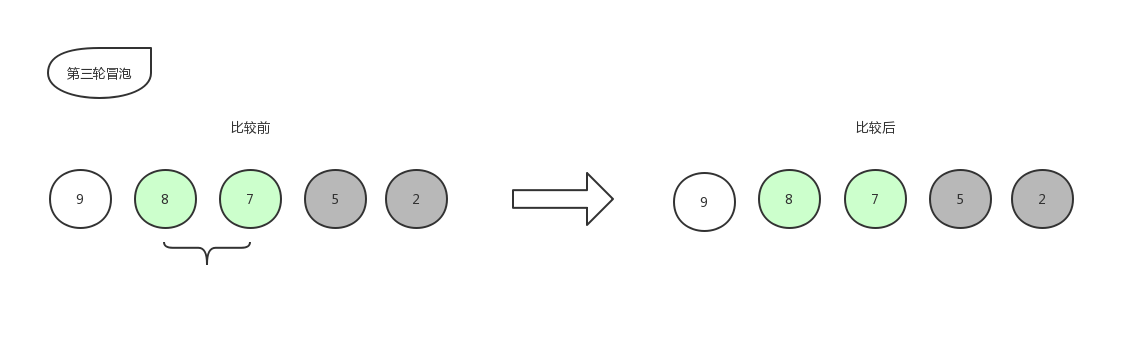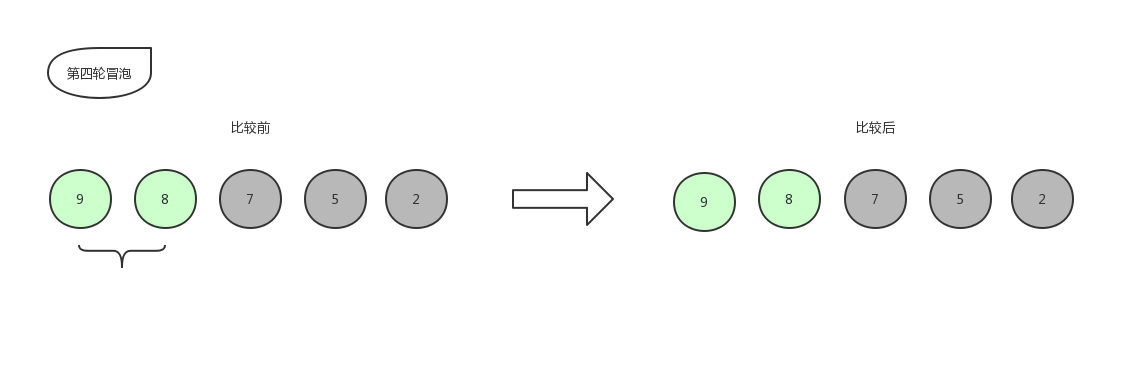9 和 8 比，位置不变，即确定了 8 进入有序序列，那么最后只剩下一个数字 9 ，放在末尾，自此排序结束。

代码实现

public static void sort(int arr[]){
for( int i = 0 ; i < arr.length - 1 ; i++ ){
for(int j = 0;j < arr.length - 1 - i ; j++){
int temp = 0;
if(arr[j] < arr[j + 1]){
temp = arr[j];
arr[j] = arr[j + 1];
arr[j + 1] = temp;
}
}
}
}

冒泡优化

public static void sort(int arr[]){
for( int i = 0;i < arr.length - 1 ; i++ ){
boolean isSort = true;
for( int j = 0;j < arr.length - 1 - i ; j++ ){
int temp = 0;
if(arr[j] < arr[j + 1]){
temp = arr[j];
arr[j] = arr[j + 1];
arr[j + 1] = temp;
isSort = false;
}
}
if(isSort){
break;
}
}
}

选择排序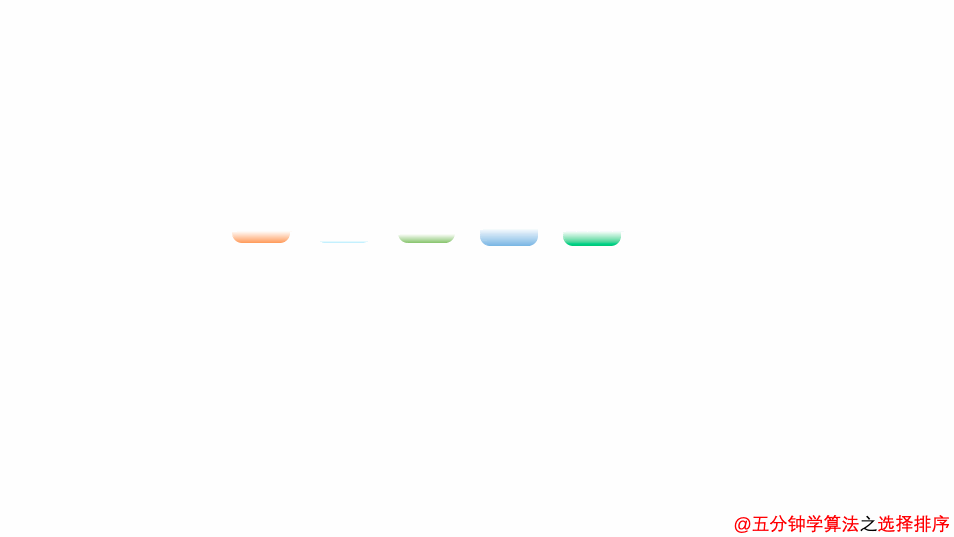图解选排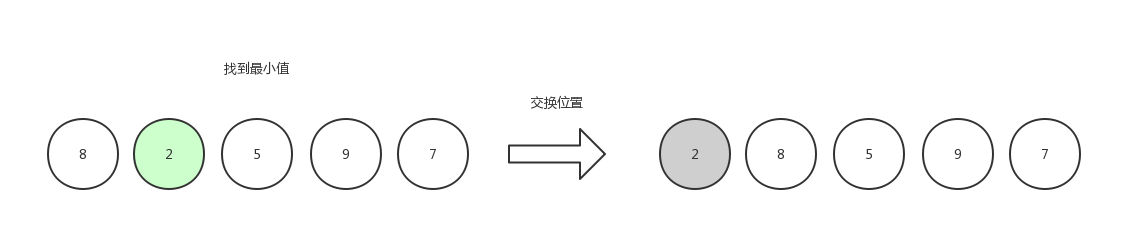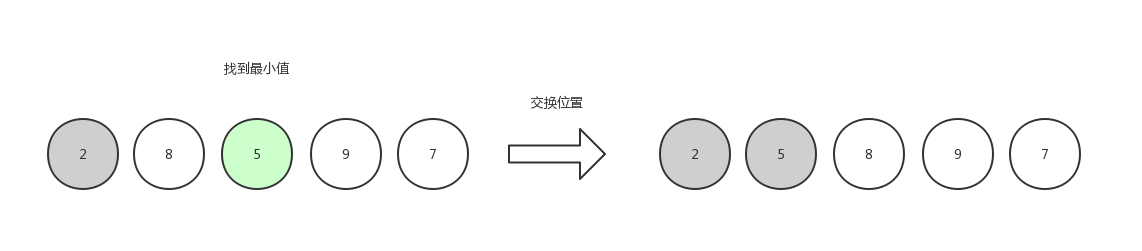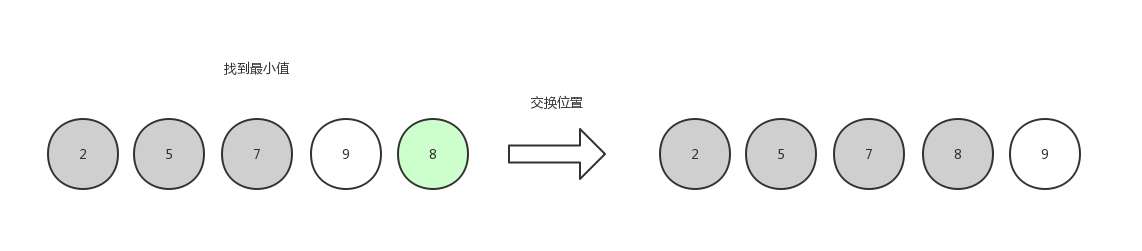代码实现

public static void sort(int arr[]){
for( int i = 0;i < arr.length ; i++ ){
int min = i;//最小元素的下标
for(int j = i + 1;j < arr.length ; j++ ){
if(arr[j] < arr[min]){
min = j;//找最小值
}
}
//交换位置
int temp = arr[i];
arr[i] = arr[min];
arr[min] = temp;
}
}

插入排序图解插排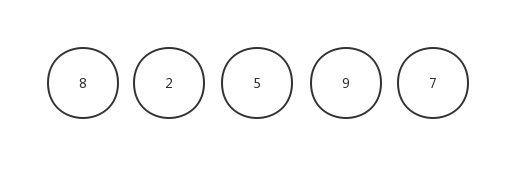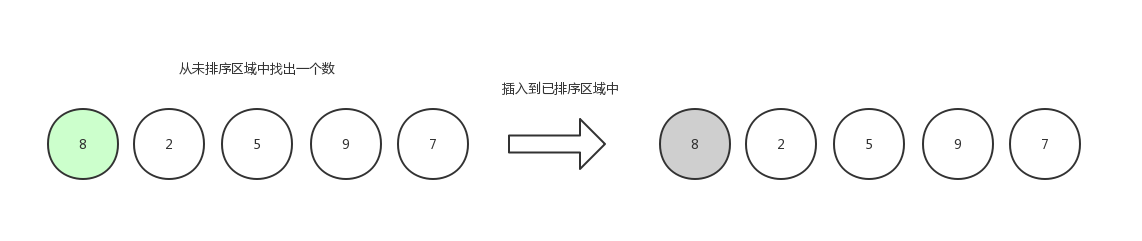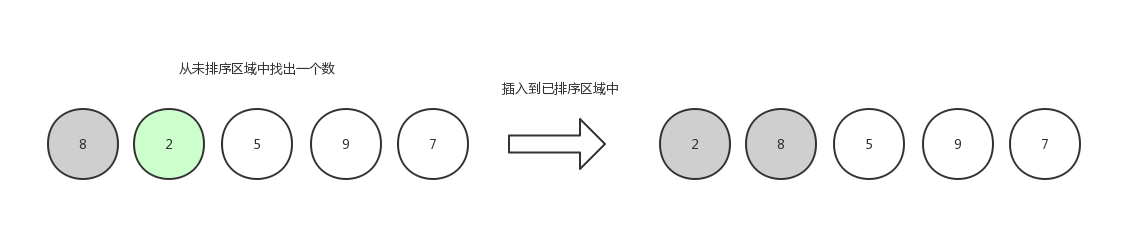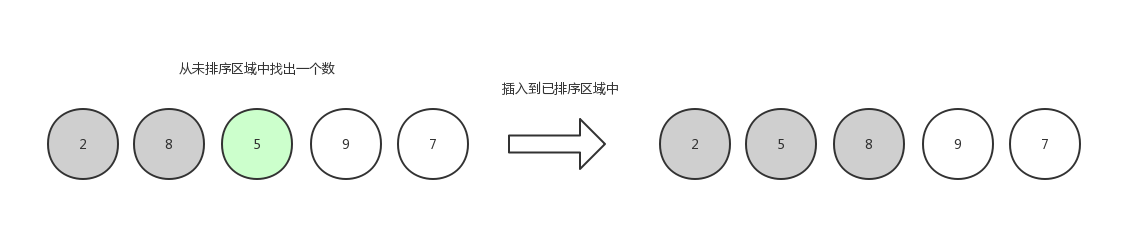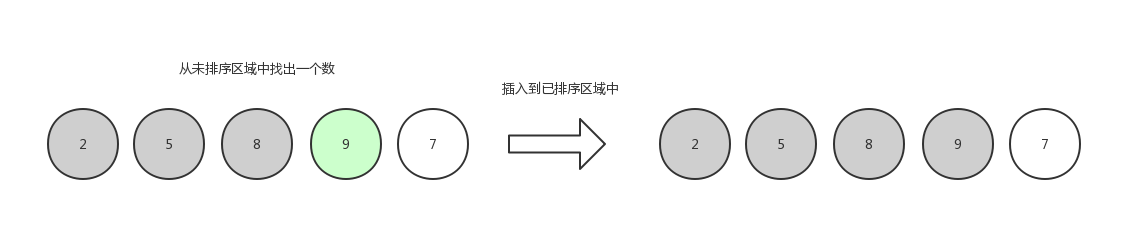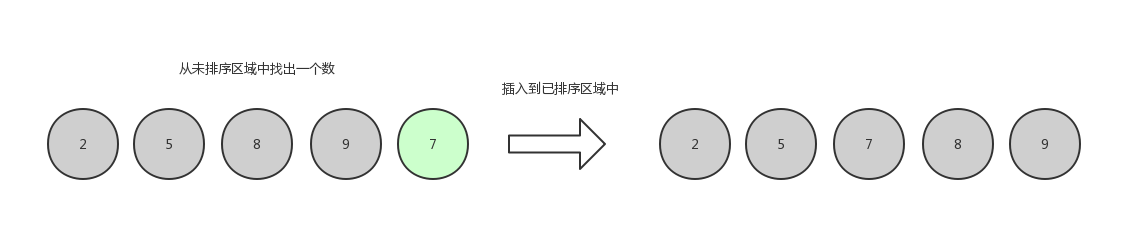代码实现

public static void sort(int[] arr) {
int n = arr.length;
for (int i = 1; i < n; ++i) {
int value = arr[i];
int j = 0;//插入的位置
for (j = i-1; j >= 0; j--) {
if (arr[j] > value) {
arr[j+1] = arr[j];//移动数据
} else {
break;
}
}
arr[j+1] = value; //插入数据
}
}

希尔排序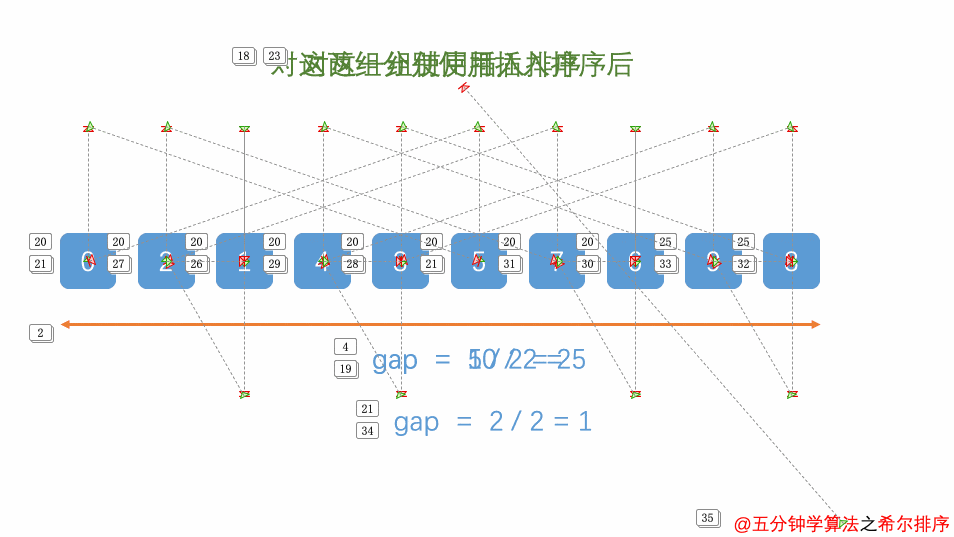图解希尔排序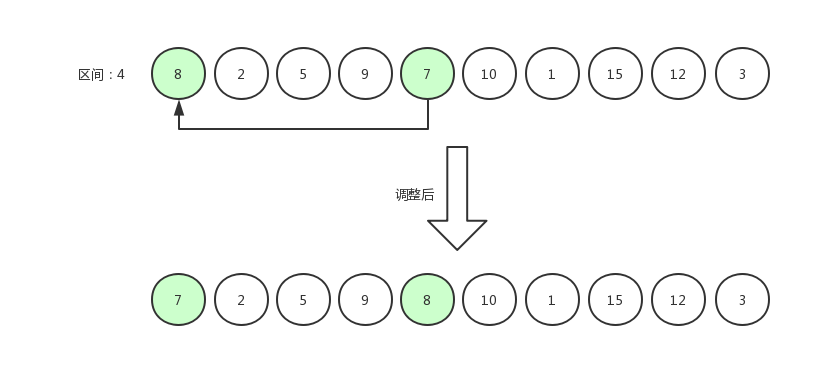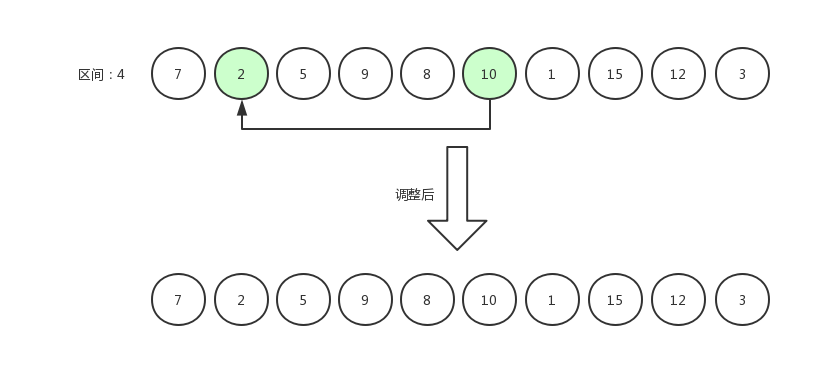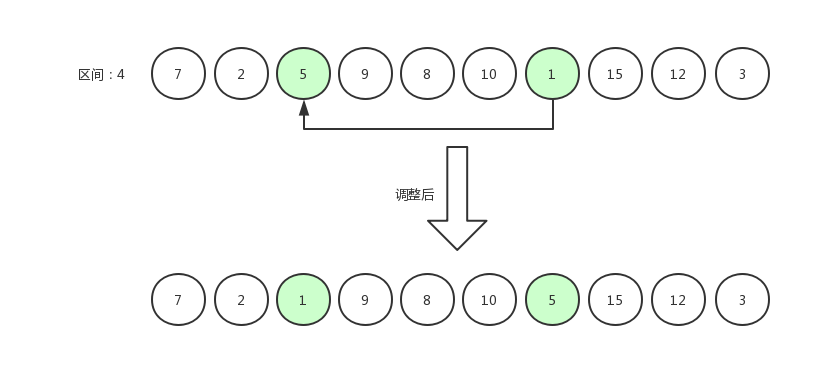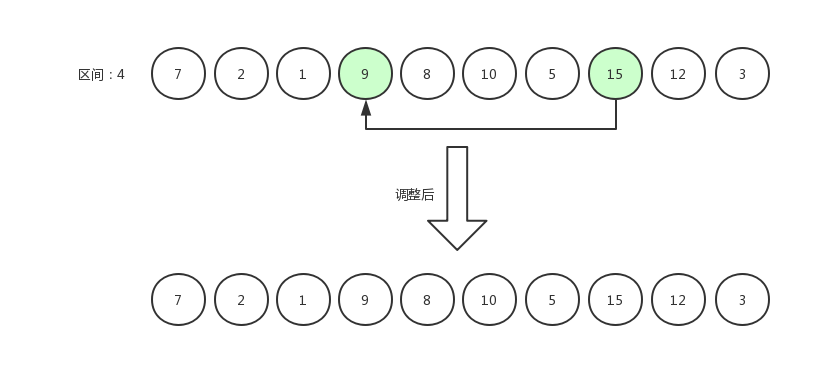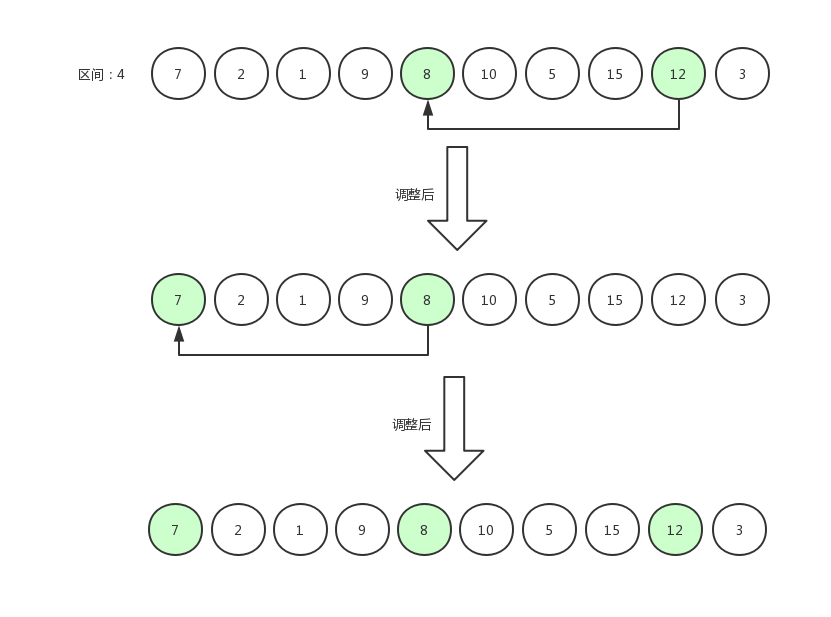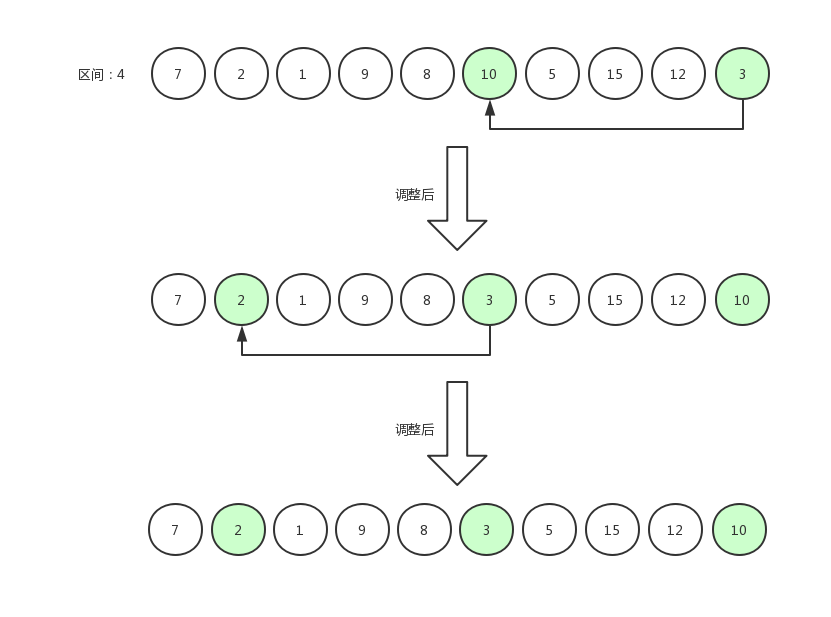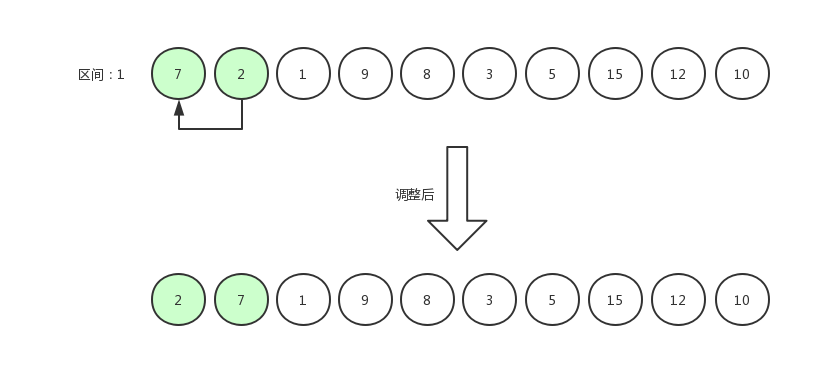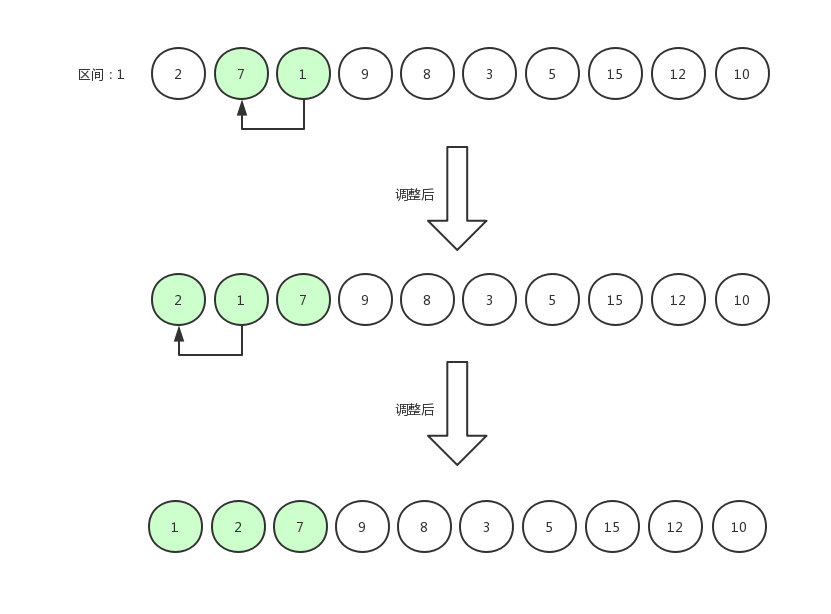代码实现

public static void sort(int[] arr) {
int length = arr.length;
//区间
int gap = 1;
while (gap < length) {
gap = gap * 3 + 1;
}
while (gap > 0) {
for (int i = gap; i < length; i++) {
int tmp = arr[i];
int j = i - gap;
//跨区间排序
while (j >= 0 && arr[j] > tmp) {
arr[j + gap] = arr[j];
j -= gap;
}
arr[j + gap] = tmp;
}
gap = gap / 3;
}
}

归并排序图解归并排序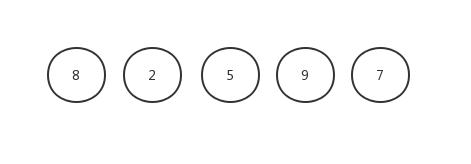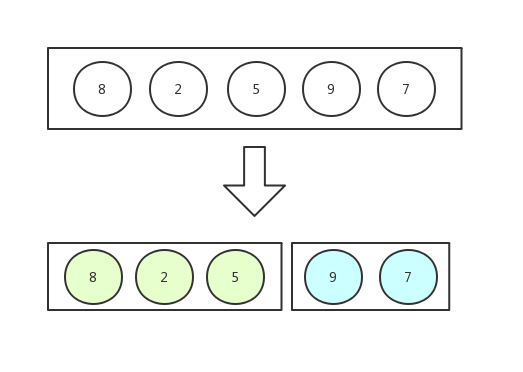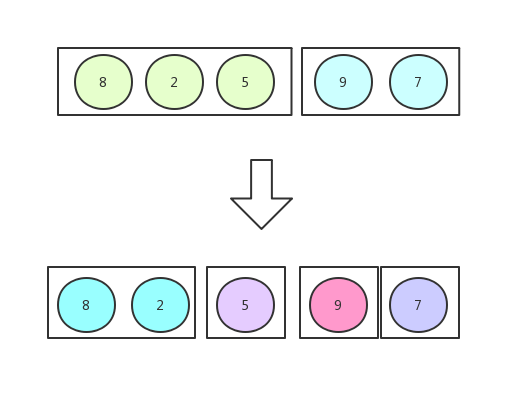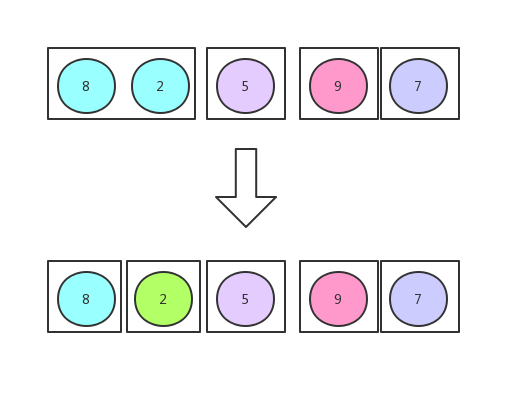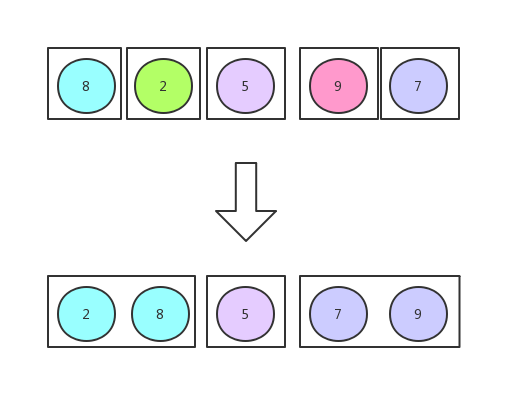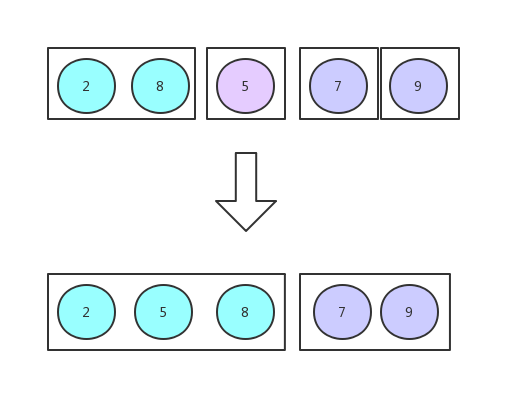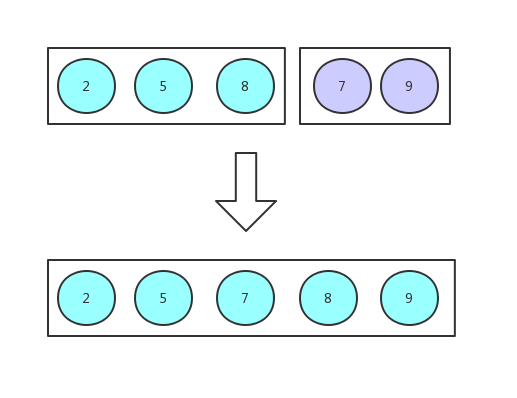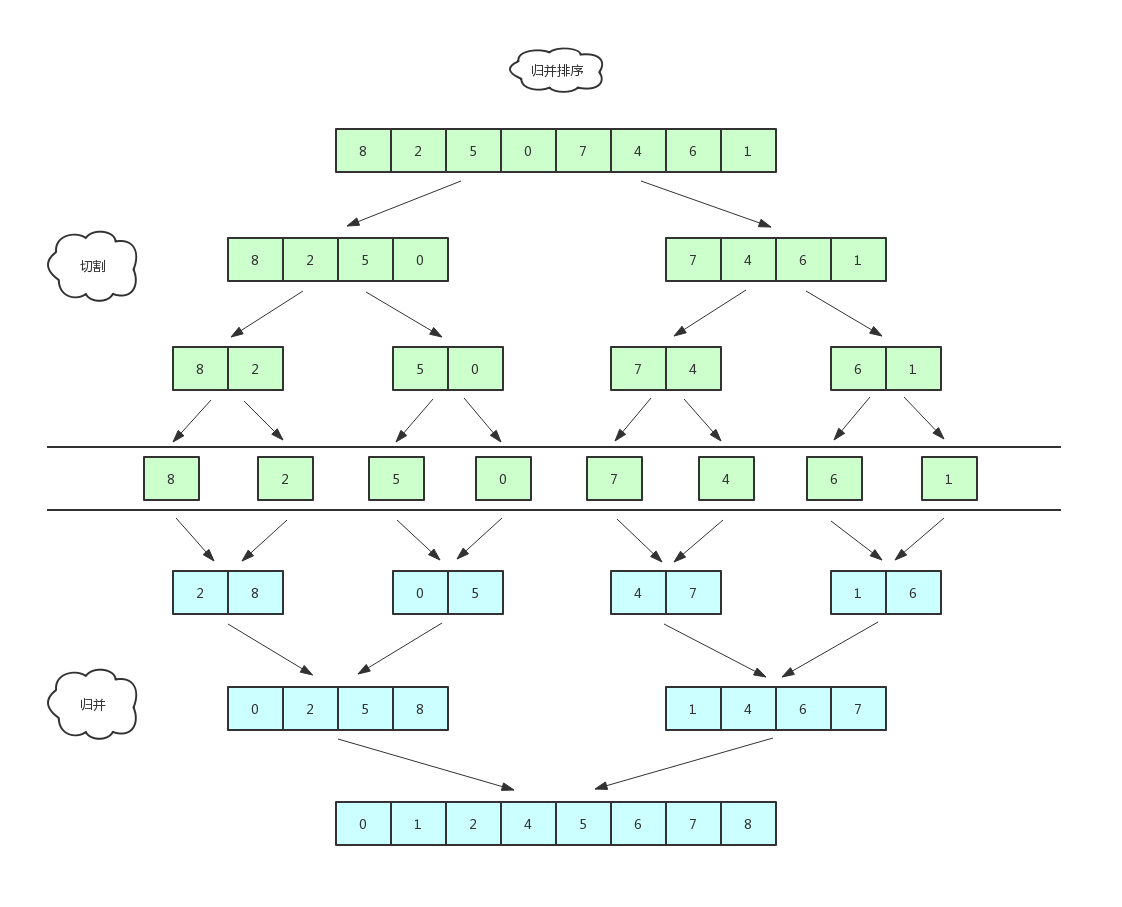代码实现

    public static void sort(int[] arr) {
int[] tempArr = new int[arr.length];
sort(arr， tempArr， 0， arr.length-1);
}

/**
* 归并排序
* @param arr 排序数组
* @param tempArr 临时存储数组
* @param startIndex 排序起始位置
* @param endIndex 排序终止位置
*/
private static void sort(int[] arr，int[] tempArr，int startIndex，int endIndex){
if(endIndex <= startIndex){
return;
}
//中部下标
int middleIndex = startIndex + (endIndex - startIndex) / 2;

//分解
sort(arr，tempArr，startIndex，middleIndex);
sort(arr，tempArr，middleIndex + 1，endIndex);

//归并
merge(arr，tempArr，startIndex，middleIndex，endIndex);
}

/**
* 归并
* @param arr 排序数组
* @param tempArr 临时存储数组
* @param startIndex 归并起始位置
* @param middleIndex 归并中间位置
* @param endIndex 归并终止位置
*/
private static void merge(int[] arr， int[] tempArr， int startIndex， int middleIndex， int endIndex) {
//复制要合并的数据
for (int s = startIndex; s <= endIndex; s++) {
tempArr[s] = arr[s];
}

int left = startIndex;//左边首位下标
int right = middleIndex + 1;//右边首位下标
for (int k = startIndex; k <= endIndex; k++) {
if(left > middleIndex){
//如果左边的首位下标大于中部下标，证明左边的数据已经排完了。
arr[k] = tempArr[right++];
} else if (right > endIndex){
//如果右边的首位下标大于了数组长度，证明右边的数据已经排完了。
arr[k] = tempArr[left++];
} else if (tempArr[right] < tempArr[left]){
arr[k] = tempArr[right++];//将右边的首位排入，然后右边的下标指针+1。
} else {
arr[k] = tempArr[left++];//将左边的首位排入，然后左边的下标指针+1。
}
}
}

快速排序图解快排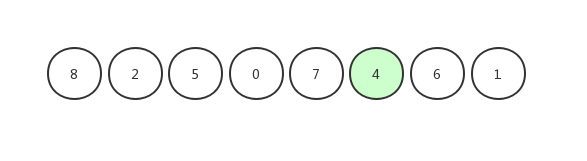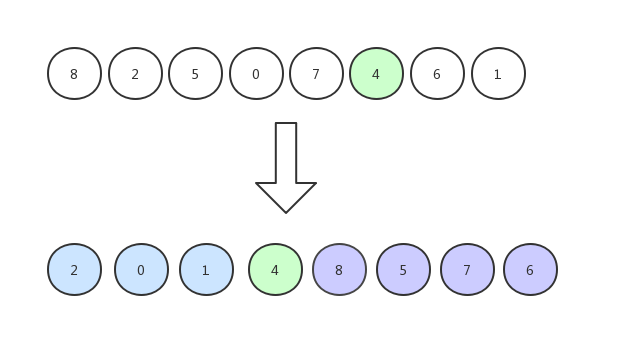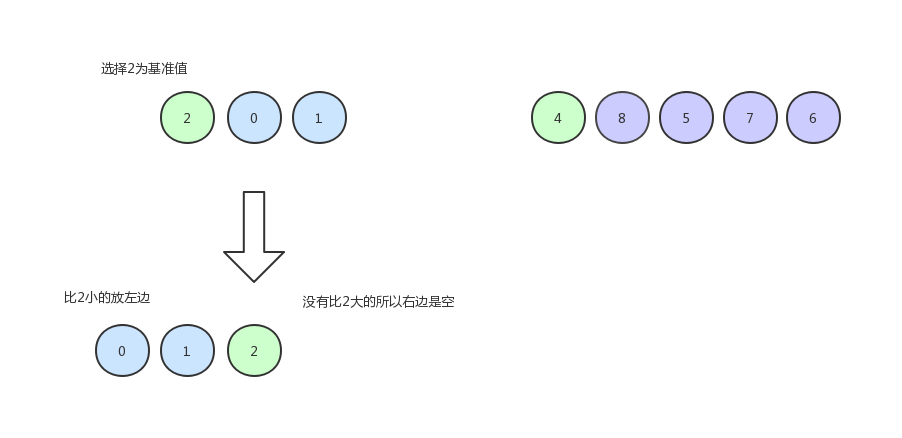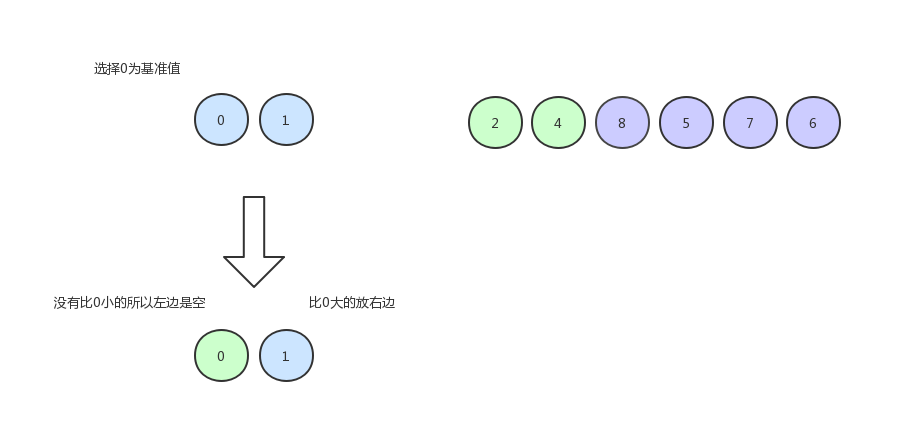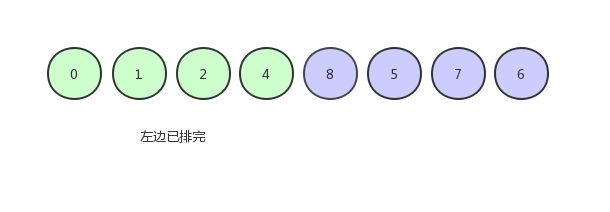单边扫描

代码实现：

public static void sort(int[] arr) {
sort(arr， 0， arr.length - 1);
}

private static void sort(int[] arr， int startIndex， int endIndex) {
if (endIndex <= startIndex) {
return;
}
//切分
int pivotIndex = partitionV2(arr， startIndex， endIndex);
sort(arr， startIndex， pivotIndex-1);
sort(arr， pivotIndex+1， endIndex);
}

private static int partition(int[] arr， int startIndex， int endIndex) {
int pivot = arr[startIndex];//取基准值
int mark = startIndex;//Mark初始化为起始下标

for(int i=startIndex+1; i<=endIndex; i++){
if(arr[i]<pivot){
//小于基准值 则mark+1，并交换位置。
mark ++;
int p = arr[mark];
arr[mark] = arr[i];
arr[i] = p;
}
}
//基准值与mark对应元素调换位置
arr[startIndex] = arr[mark];
arr[mark] = pivot;
return mark;
}

双边扫描

`
public static void sort(int[] arr) {
sort(arr， 0， arr.length - 1);
}